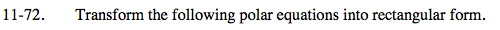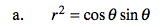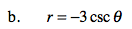### Home > PC > Chapter 11 > Lesson 11.2.1 > Problem11-72

11-72.Multiply both sides of the equation by r2.

$\textit{r}^4=(\textit{r}\cos\theta)(\textit{r}\sin\theta)$

Substitute the polar coordinate conversions.

(x2 + y2)2 = xyMultiply both sides of the equation by sin θ.